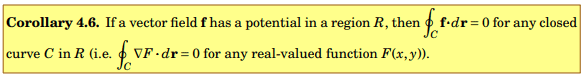# Line Integral question

• A
• WMDhamnekar

#### WMDhamnekar

MHB
TL;DR Summary
Let g(x) and h(y) be differentiable functions, and let f(x, y) = h(y)i+ g(x)j. Can f have a potential F(x, y)? If so, find it.

You may assume that F would be smooth.
(Hint: Consider the mixed partial derivatives of F.)
I don't have any idea about how to use the hint given by the author.
Author has given the answer to this question i-e F(x,y) = axy + bx + cy +d.
I don't understand how did the author compute this answer.

Would any member of Physics Forums enlighten me in this regard?

Any math help will be accepted.

Because
## - \dfrac{\partial F}{\partial x} = h(y) ##
then
## \dfrac{\partial^2 F}{\partial x^2} = 0 ##

And we also have
##\dfrac{\partial^2 F}{\partial x \partial y } = \dfrac{\partial^2 F}{\partial y \partial x } ##

If you just want to show that the potential exists, then show that ##\vec \nabla \times \vec f = 0 ##

Last edited by a moderator:
•WMDhamnekar
Because
## - \dfrac{\partial F}{\partial x} = h(y) ##
then
## \dfrac{\partial^2 F}{\partial x^2} = 0 ##

And we also have
##\dfrac{\partial^2 F}{\partial x \partial y } = \dfrac{\partial^2 F}{\partial y \partial x } ##
Why is the sign negative for ## \frac{\partial{F}}{\partial{x}}= h(y)?## Would you explain that ?

Why is the sign negative for ## \frac{\partial{F}}{\partial{x}}= h(y)?## Would you explain that ?

Isn't the potential F(x,y) defined as ##\vec f = - \vec \nabla F## ?

•WMDhamnekar
Isn't the potential F(x,y) defined as ##\vec f = - \vec \nabla F## ?
In the following corollary 4.6 ## \vec f \not= -\vec \nabla F ## Why?In the following corollary 4.6 ## \vec f \not= -\vec \nabla F ## Why?
View attachment 303550

Matter of convetion I guess, I have always used ##\vec f = - \vec \nabla F## as the definition as potential. But it does not matter, the math is the same, just follow your books conventions. And also -0 = 0 so...

Last edited by a moderator: